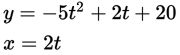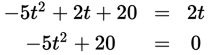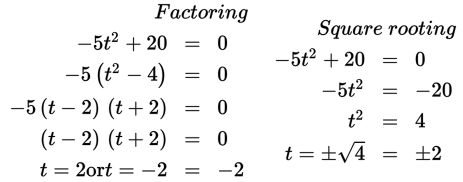# SAT Math Multiple Choice Question 935: Answer and Explanation

### Test Information

Question: 9353. A cannonball is shot out of a cannon at a 45° angle with an approximate speed of 283m/s. The cannon from which the ball was fired sits on the edge of a cliff, and itsheight above the ground is 20 meters. The equations given above represent the cannonball'sheight above the ground (y) and its horizontal distance (x) from the face of the cliff, t seconds after it was fired, where t ≥ 0. How many seconds after the ball was fired does its vertical height above theground equal its horizontal distance from the cliff?

• A. 1
• B. 2
• C. 3
• D. 4

Explanation:

B

Difficulty: Medium